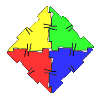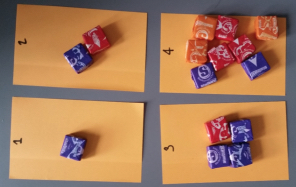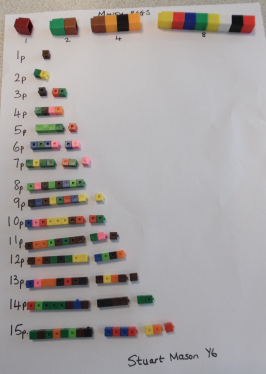#### You may also like### Geoboards

This practical challenge invites you to investigate the different squares you can make on a square geoboard or pegboard.### Polydron

This activity investigates how you might make squares and pentominoes from Polydron.If you had 36 cubes, what different cuboids could you make?

# Money Bags

##### Age 5 to 11Challenge Level
We had quite a few submissions for this challenge and these make interesting reading.

Harrison School sent in a solution from  Charlie and Oscar as follows;

To begin, we decided to use post-it-notes as bags and sweets to represent our pennies - this made it easy to visualise the question. Then, we worked out that one could only be added to zero in order to make itself; there was now a bag with one penny in it. After that, we made a bag with two pennies in. We did think that we could make two with two ones yet that would give us a problem later. Next, we worked out that three could already be made using bags one and two. However, four could not be made with bags one and two, therefore we had to make bag three have four pennies in it. We then looked and saw we could make numbers five (bag one and three), six (bags three and two) and seven (bags one, two and three) but eight was unable to be made. Consequently, that means that eight has to be in bag four. This used up all the pennies - there were none left over. Finally, we checked that the numbers nine to fifteen worked, which they did. Nine = bags four and one. Ten = bags four and two. Eleven = bags four, two and one. Twelve = bags four and three. Thirteen = bags four, three and one. Fourteen = bags four, three and two. Fifteen = bags four, three, two and one.Uma from Westhill Primary School, Aberdeenshire sent in her thoughts and the solution.

The solution for the Money Bags problem is 1,2,4 and 8 Here is the explanation of how I got my answer:
There are four bags so there can only be four different types of coins. The basic idea behind the solution is to find the four different numbers which can add up to give 1p to 15p. The solution for the question is 1,2,4 and 8. The 1st bag holds 1x1p, the 2nd bag contains 2x1p, the 3rd bag holds 4x1p and the 4th bag contains 8x1p. We can get any number between 1 to 15 using any combination of 1,2,4 and 8.

1p = Bag 1;  2p = Bag 2;  3p = Bag 1 + Bag 2;  4p = Bag 3;  5p = Bag 3 + Bag 1
6p = Bag 3 + Bag 2;  7p = Bag 3 + Bag 2 + Bag 1;  8p = Bag 4;  9p = Bag 4 + Bag 1
10p = Bag 4 + Bag 2;  11p = Bag 4 + Bag 2 + Bag 1;  12p = Bag 4 + Bag 3;
13p = Bag 4 + Bag 3 + Bag 1;  14p = Bag 4 + Bag 3 + Bag 2
15p = Bag 4 + Bag 3 + Bag 2 + Bag 1

Mr Howes' Magnificent Maths Group from  Pierrepont Gamston Primary School

As part of an intervention group, we were a little confused to start off with - we didn't realise that the question was asking us to split the money into four bags so that the bags' totals could be combined to make any value up to 15p. Once we worked this out we wrote the values 1p to 15p down the side of the page and tried to find a system to solving the problem.

1p = this was easy, it had to be made with a bag with 1p in it!
2p = this could be made by another bag having 1p in it, or a second bag having 2p in it. We  went for the latter because it stopped us using up our bags too quickly!
3p = this was also easy: 1p+2p
4p = We could either have another 1p or 2p bag, or a new 4p bag. We used the 4p bag.

At this point we spotted a pattern - 1p, 2p, 4p... would the next bag contain 8p?

5p = 4p+1p;  6p = 4p+2p;  7p = 4p+2p+1p;  8p = We stuck to our prediction and put 8p in the final bag!;  9p = 8p+1p;  10p = 8p+2p;  11p = 8p+2p+1p;  12p = 8p +4p;  13p = 8p+4p+1p
14p = 8p+4p+2p;  15p = 8p+4p+2p+1p

We thought that if the pattern was continued, the next bag should contain 16p ( making the total value 29p).
We wondered if this would be a better system for the values of coins; however, we realised that the values we used help us add up the numbers mentally much faster and make finding change easier!
James, Elliot, Freddie, Anna, Orla and Oliver

Jake from Duke of Norfolk School said

We drew out circles to show the different bags.

We tried to make all the numbers from 1 to 15, and recorded the number of pennies used in the circles.
We started with 1, 2, 3 and 5 coins, but found that we could not make numbers up from 12. We realised that we had to keep 1 and 2, because they were needed to pay 1p and 2p. So we added coins to the other bags, eventually settling on 1, 2, 4 and 8.

Stuart from Dussindale Primary School sent in this work and a good picture illustrating the solution

I started to look at multiples of ten but then realised I needed to use doubles/halves.
My first pair were 2 and 8 and then I saw I needed to have 1 and 2 and 4 and 8.
I used the cubes to make sure that I could make all the numbers from 1 to 15.Sarah from Cabot Primary School, Bristol sent in the following;

A Y4 maths group from Cabot Primary School, Bristol (Iman, Andrea-Marie, Sakariya and Aisha) worked on this problem together.
First of all, we found 15 play coins and a painting tray to help us think about the investigation and find a solution to the maths problem.
Secondly, we decided that we definitely needed to have one bag with 1p in it (or we would not be able to pay for something that cost 1p!), so we put 1p in one of the pots.

Then we thought about which numbers could make all of the other prices up to 15p. We realised that if we also had a bag with 2p in it, we could make 2p and 3p (1p + 2p), so we put 2p in our second pot.  We tried 3p in the third pot and this gave us more prices:
1p (1p);  2p (2p);  3p (3p);  4p (3p + 1p);  5p (3p + 2p);  6p (3p + 2p + 1p)
...but we couldn't make 7p.

So we tried again, this time with 4p in the third pot.  We could then make all of the prices up to 7p.
1p (1p);  2p (2p);  3p (2p + 1p);  4p (4p);  5p (4p + 1p);  6p (4p + 2p);  7p (4p + 2p + 1p).

We only had one empty pot left, so we put all of the rest of the coins into it - there were 8 of them.  We then found that you could make all of the other numbers:
8p (8p);  9p (8p + 1p);  10p (8p + 2p);  11p (8p + 2p + 1p);  12p (8p + 4p);  13p (8p + 4p + 1p);   14p (8p + 4p + 2p);  15p (8p + 4p + 2p + 1p)

So we knew we had found the solution!  We tried all the possibilities and didn't give up because Cabot Primary children are resilient!  PS Our paint tray actually has six pots in it.  We can see a pattern building up and are now going to think about how to investigate the maximum number of prices we can make if we use all six pots.

Later Sarah wrote and sent in this;

When we looked again at the number of coins in each pot, we noticed that each number was double the previous one.  Since double 8 is 16, we put 16 coins into the next empty pot so we had five pots containing 1p, 2p, 4p, 8p and 16p. We knew the maximum price we could now pay would be 31p (our existing 15p plus the extra pot of 16p). We checked that we could now make all of the prices from 15p to 31p (which we could - by adding the new pot of money to each of the combinations that we had already found).  We knew that we had found a system and that the sixth pot should therefore contain 32p (which is double 16p) and that we would  be able to make all of the prices up to a maximum of 63p.

We then came up with this sequence to show how much money twelve pots (two paint trays) would contain:
1p,    2p,    4p,    8p,    16p,    32p,    64p,    £1.28,    £2.56,    £5.12,     £10.24     £20.48

It was good to hear about the different ways that solutions were found. Well done all  of you.